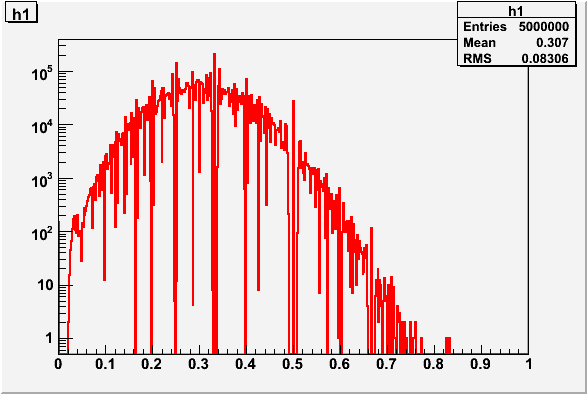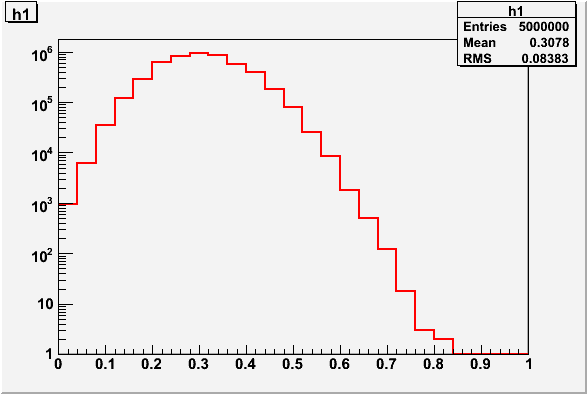# Simulation with TRandom

Dear ROOT experts,

If you look at the attached plot produced by the attached macro trySimulation.C, there is
a dip around 0.5, which presents there all the time? Could you tell me how to avoid that?
The root version I use is 5.15/08.

Best regards,
Zhongbao
trySimulation.C (612 Bytes)

Hello,

the problem is due to a bin effect of the histogram and the fact that you are generating discrete events.
Either use an integer number in the abscissa of the histogram (like from 0 to nmax) or smear the number by +/- 1 by doing for example:

``````    Int_t n1 = rand.Binomial(n, fp);

h1->Fill((Double_t(n1)+ (rand.Rndm()-0.5))/n);``````

Best Regards

Lorenzo

Damn, somewhat beaten, concisely and a better fix, too.[/edit]

It seems that this problem is intrinsic to the way you have constructed your distribution.

You’re filling the histogram with (integer1) / (integer2) where ~30 < integer1 ~55 and ~5 < integer2 < ~25 (Just looking at a sample of ~50 numbers) - I think it seems reasonably obvious that there are some real numbers that you cannot produce with any combination of integers in that range. You can see this if you increase the number of bins in the histogram - You get some bins with 0 entries (See first attachment).